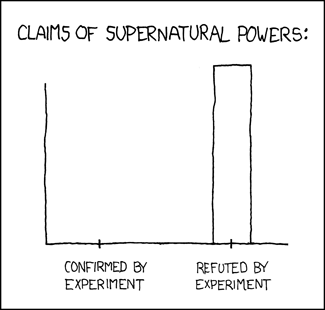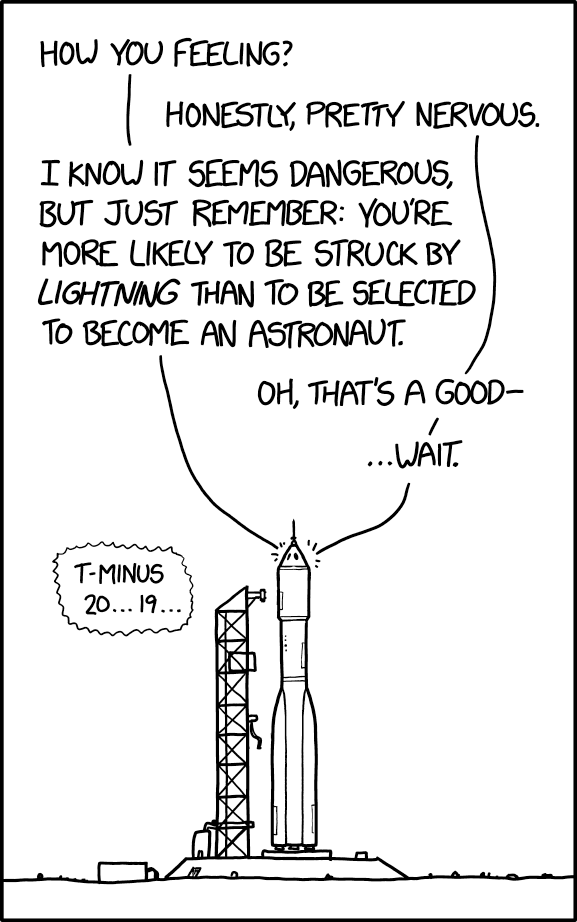## leave Bayes factors where they once belonged

Posted in Statistics with tags , , , , , , , , , , on February 19, 2019 by xi'anIn the past weeks I have received and read several papers (and X validated entries)where the Bayes factor is used to compare priors. Which does not look right to me, not on the basis of my general dislike of Bayes factors!, but simply because this seems to clash with the (my?) concept of Bayesian model choice and also because data should not play a role in that situation, from being used to select a prior, hence at least twice to run the inference, to resort to a single parameter value (namely the one behind the data) to decide between two distributions, to having no asymptotic justification, to eventually favouring the prior concentrated on the maximum likelihood estimator. And more. But I fear that this reticence to test for prior adequacy also extends to the prior predictive, or Box’s p-value, namely the probability under this prior predictive to observe something “more extreme” than the current observation, to quote from David Spiegelhalter.## Posterior predictive p-values and the convex order

Posted in Books, Statistics, University life with tags , , , , , , , , , on December 22, 2014 by xi'an

Patrick Rubin-Delanchy and Daniel Lawson [of Warhammer fame!] recently arXived a paper we had discussed with Patrick when he visited Andrew and I last summer in Paris. The topic is the evaluation of the posterior predictive probability of a larger discrepancy between data and model$\mathbb{P}\left( f(X|\theta)\ge f(x^\text{obs}|\theta) \,|\,x^\text{obs} \right)$

which acts like a Bayesian p-value of sorts. I discussed several times the reservations I have about this notion on this blog… Including running one experiment on the uniformity of the ppp while in Duke last year. One item of those reservations being that it evaluates the posterior probability of an event that does not exist a priori. Which is somewhat connected to the issue of using the data “twice”.

“A posterior predictive p-value has a transparent Bayesian interpretation.”

Another item that was suggested [to me] in the current paper is the difficulty in defining the posterior predictive (pp), for instance by including latent variables$\mathbb{P}\left( f(X,Z|\theta)\ge f(x^\text{obs},Z^\text{obs}|\theta) \,|\,x^\text{obs} \right)\,,$

which reminds me of the multiple possible avatars of the BIC criterion. The question addressed by Rubin-Delanchy and Lawson is how far from the uniform distribution stands this pp when the model is correct. The main result of their paper is that any sub-uniform distribution can be expressed as a particular posterior predictive. The authors also exhibit the distribution that achieves the bound produced by Xiao-Li Meng, Namely that$\mathbb{P}(P\le \alpha) \le 2\alpha$

where P is the above (top) probability. (Hence it is uniform up to a factor 2!) Obviously, the proximity with the upper bound only occurs in a limited number of cases that do not validate the overall use of the ppp. But this is certainly a nice piece of theoretical work.

## ABC model choice by random forests

Posted in pictures, R, Statistics, Travel, University life with tags , , , , , , , , , , , , , on June 25, 2014 by xi'anAfter more than a year of collaboration, meetings, simulations, delays, switches,  visits, more delays, more simulations, discussions, and a final marathon wrapping day last Friday, Jean-Michel Marin, Pierre Pudlo,  and I at last completed our latest collaboration on ABC, with the central arguments that (a) using random forests is a good tool for choosing the most appropriate model and (b) evaluating the posterior misclassification error rather than the posterior probability of a model is an appropriate paradigm shift. The paper has been co-signed with our population genetics colleagues, Jean-Marie Cornuet and Arnaud Estoup, as they provided helpful advice on the tools and on the genetic illustrations and as they plan to include those new tools in their future analyses and DIYABC software.  ABC model choice via random forests is now arXived and very soon to be submitted…One scientific reason for this fairly long conception is that it took us several iterations to understand the intrinsic nature of the random forest tool and how it could be most naturally embedded in ABC schemes. We first imagined it as a filter from a set of summary statistics to a subset of significant statistics (hence the automated ABC advertised in some of my past or future talks!), with the additional appeal of an associated distance induced by the forest. However, we later realised that (a) further ABC steps were counterproductive once the model was selected by the random forest and (b) including more summary statistics was always beneficial to the performances of the forest and (c) the connections between (i) the true posterior probability of a model, (ii) the ABC version of this probability, (iii) the random forest version of the above, were at best very loose. The above picture is taken from the paper: it shows how the true and the ABC probabilities (do not) relate in the example of an MA(q) model… We thus had another round of discussions and experiments before deciding the unthinkable, namely to give up the attempts to approximate the posterior probability in this setting and to come up with another assessment of the uncertainty associated with the decision. This led us to propose to compute a posterior predictive error as the error assessment for ABC model choice. This is mostly a classification error but (a) it is based on the ABC posterior distribution rather than on the prior and (b) it does not require extra-computations when compared with other empirical measures such as cross-validation, while avoiding the sin of using the data twice!

Posted in Books, Statistics, Travel, University life with tags , , , , , , , on September 3, 2013 by xi'an

Today, I attended the RSS Annual Conference in Newcastle-upon-Tyne. For one thing, I ran a Memorial session in memory of George Casella, with my (and his) friends Jim Hobert and Elias Moreno as speakers. (The session was well-attended if not overwhelmingly so.) For another thing, the RSS decided to have the DIC Read Paper by David Spiegelhalter, Nicky Best, Brad Carlin and Angelika van der Linde Bayesian measures of model complexity and fit re-Read, and I was asked to re-discuss the 2002 paper. Here are the slides of my discussion, borrowing from the 2006 Bayesian Analysis paper with Gilles Celeux, Florence Forbes, and Mike Titterington where we examined eight different versions of DIC for mixture models. (I refrained from using the title “snow white and the seven DICs” for a slide…) I also borrowed from our recent discussion of Murray Aitkin’s (2009) book. The other discussant was Elias Moreno, who focussed on consistency issues. (More on this and David Spiegelhalter’s defence in a few posts!) This was the first time I was giving a talk on a basketball court (I once gave an exam there!)

## on using the data twice…

Posted in Books, Statistics, University life with tags , , , , , , , , on January 13, 2012 by xi'an

As I was writing my next column for CHANCE, I decided I will include a methodology box about “using the data twice”. Here is the draft. (The second part is reproduced verbatim from an earlier post on Error and Inference.)

Several aspects of the books covered in this CHANCE review [i.e., Bayesian ideas and data analysis, and Bayesian modeling using WinBUGS] face the problem of “using the data twice”. What does that mean? Nothing really precise, actually. The accusation of “using the data twice” found in the Bayesian literature can be thrown at most procedures exploiting the Bayesian machinery without actually being Bayesian, i.e.~which cannot be derived from the posterior distribution. For instance, the integrated likelihood approach in Murray Aitkin’s Statistical Inference avoids the difficulties related with improper priors πi by first using the data x to construct (proper) posteriors πii|x) and then secondly using the data in a Bayes factor$\int_{\Theta_1}f_1(x|\theta_1) \pi_1(\theta_1|x)\,\text{d}\theta_1\bigg/ \int_{\Theta_2}f_2(x|\theta_2)\pi_2(\theta_2|x)\,\text{d}\theta_2$

as if the posteriors were priors. This obviously solves the improperty difficulty (see. e.g., The Bayesian Choice), but it creates a statistical procedure outside the Bayesian domain, hence requiring a separate validation since the usual properties of Bayesian procedures do not apply. Similarly, the whole empirical Bayes approach falls under this category, even though some empirical Bayes procedures are asymptotically convergent. The pseudo-marginal likelihood of Geisser and Eddy (1979), used in  Bayesian ideas and data analysis, is defined by$\hat m(x) = \prod_{i=1}^n f_i(x_i|x_{-i})$

through the marginal posterior likelihoods. While it also allows for improper priors, it does use the same data in each term of the product and, again, it is not a Bayesian procedure.

Once again, from first principles, a Bayesian approach should use the data only once, namely when constructing the posterior distribution on every unknown component of the model(s).  Based on this all-encompassing posterior, all inferential aspects should be the consequences of a sequence of decision-theoretic steps in order to select optimal procedures. This is the ideal setting while, in practice,  relying on a sequence of posterior distributions is often necessary, each posterior being a consequence of earlier decisions, which makes it the result of a multiple (improper) use of the data… For instance, the process of Bayesian variable selection is on principle clean from the sin of “using the data twice”: one simply computes the posterior probability of each of the variable subsets and this is over. However, in a case involving many (many) variables, there are two difficulties: one is about building the prior distributions for all possible models, a task that needs to be automatised to some extent; another is about exploring the set of potential models. First, ressorting to projection priors as in the intrinsic solution of Pèrez and Berger (2002, Biometrika, a much valuable article!), while unavoidable and a “least worst” solution, means switching priors/posteriors based on earlier acceptances/rejections, i.e. on the data. Second, the path of models truly explored by a computational algorithm [which will be a minuscule subset of the set of all models] will depend on the models rejected so far, either when relying on a stepwise exploration or when using a random walk MCMC algorithm. Although this is not crystal clear (there is actually plenty of room for supporting the opposite view!), it could be argued that the data is thus used several times in this process…